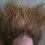# Set theory problem - closed subsets of irrationals?

Definition: A set X is closed under addition if, for p and q both in X (with p and q not necessarily distinct!), p+q is always in X.

Easy question: Is there a non-empty subset of the irrationals that is closed under addition?

Tricky question? Is there an uncountably infinite non-empty subset of the irrationals that is closed under addition?

For example, the set {sqrt(2)} fails because it is not closed; taking p=sqrt(2), q=sqrt(2), we find that p+q=2*sqrt(2) is not in the set. The set of reals fails because it is not a subset of the irrationals.

I came up with this problem today, solved it, and I hope it makes you think. :)Note by Alex Meiburg
6 years, 8 months ago

This discussion board is a place to discuss our Daily Challenges and the math and science related to those challenges. Explanations are more than just a solution — they should explain the steps and thinking strategies that you used to obtain the solution. Comments should further the discussion of math and science.

When posting on Brilliant:

• Use the emojis to react to an explanation, whether you're congratulating a job well done , or just really confused .
• Ask specific questions about the challenge or the steps in somebody's explanation. Well-posed questions can add a lot to the discussion, but posting "I don't understand!" doesn't help anyone.
• Try to contribute something new to the discussion, whether it is an extension, generalization or other idea related to the challenge.

MarkdownAppears as
*italics* or _italics_ italics
**bold** or __bold__ bold
- bulleted- list
• bulleted
• list
1. numbered2. list
1. numbered
2. list
Note: you must add a full line of space before and after lists for them to show up correctly
paragraph 1paragraph 2

paragraph 1

paragraph 2

[example link](https://brilliant.org)example link
> This is a quote
This is a quote
    # I indented these lines
# 4 spaces, and now they show
# up as a code block.

print "hello world"
# I indented these lines
# 4 spaces, and now they show
# up as a code block.

print "hello world"
MathAppears as
Remember to wrap math in $$ ... $$ or $ ... $ to ensure proper formatting.
2 \times 3 $2 \times 3$
2^{34} $2^{34}$
a_{i-1} $a_{i-1}$
\frac{2}{3} $\frac{2}{3}$
\sqrt{2} $\sqrt{2}$
\sum_{i=1}^3 $\sum_{i=1}^3$
\sin \theta $\sin \theta$
\boxed{123} $\boxed{123}$

Sort by:

The set of reals $\mathbb{R}$ is uncountably infinitely-dimensional as a vector space over $\mathbb{Q}$. Let $X$ be a Hamel basis for $\mathbb{R}$ which contains $1$, and let $Y = X \backslash \{1\}$ be everything in the Hamel basis except $1$. Certainly $Y$ is uncountable.

Let $Z$ be the set of finite sums of elements of $Y$, so that $Z$ is the set of real numbers of the form $z \; = \; n_1y_1 + n_2y_2 + \cdots + n_my_m$ where $m \in \mathbb{N}$ and $n_1,n_2,\ldots,n_m \in \mathbb{N}$, while $y_1,y_2,\ldots,y_m \in Y$. It is clear that:

1. $Z$ is uncountable, since it has $Y$ as a subset,

2. $Z$ is closed under addition,

3. No element of $Z$ is rational. If $Z \cap \mathbb{Q} \neq \varnothing$, we could find $m,n_1,n_2,\ldots,n_m \in \mathbb{N}$ and distinct $y_1,y_2,\ldots,y_m \in Y$ and $q \in \mathbb{Q}$ such that $n_1y_1 + n_2y_2 + \cdots + n_my_m - q\times1 \; = \; 0$ But this tells us that there is a nontrivial rational linear dependence relation between the elements $y_1,y_2,\ldots,y_m,1$ of $X$, which is not possible.

Thus $Z$ does the job. I would not want to attempt to write down a concrete example of a set $Z$!

- 6 years, 7 months ago

Nice! ^__^ And much better explained that I ever would have. :P

- 6 years, 7 months ago

The set with elements multiples of root 2 (only positive multiples)

- 6 years, 8 months ago

Yes, this satisfies the first one. :D It obviously does not satisfy the second one, though; I suggest trying that one too. :)

- 6 years, 8 months ago

2nd 1 is really difficult...

- 6 years, 8 months ago

I tried the 2nd one bt i could not, plz tell me the answer...

- 6 years, 8 months ago